# Forced Vibration Analysis of Functionally Graded Beams Carrying Moving Harmonic Loads under Random Boundary Conditions

Forced Vibration Analysis of Functionally Graded Beams Carrying Moving Harmonic Loads under Random Boundary Conditions
Laboratory Mechanics of Structures and Solids, Department of Mechanical Engineering, Faculty of Technology, University of Sidi Bel Abbes, Sidi Bel Abbes 22000, Algeria

Corresponding Author Email:
mechanicsgroup22@gmail.com
Page:
258-264
|
DOI:
https://doi.org/10.18280/mmep.070212
9 March 2020
|
Accepted:
26 May 2020
|
Published:
30 June 2020
| Citation

OPEN ACCESS

Abstract:

This paper introduces forced vibration analysis of functionally graded materials (FGMs) beams subjected to moving harmonic loads in different physical and geometric states, under random boundary conditions. A mathematical model was developed based on a new refined logarithmic shear deformation theory (LSBT), used the Hamilton principle combined with the introduction of weak forms into the dynamic analysis, while including rotational inertia. The raster force is designed by the Dirac-delta function expressing moving harmonic loads. The Rayleigh-Ritz solution is used to separate system variables from equations with general boundary conditions. The fundamental frequencies of free vibration analysis are determined by solving the system of equations governing the eigenvalue problems and the modal responses of forced vibration behavior are also solved numerically using Newmark's temporal integration method. The numerical results presented make it possible to clearly appreciate the contribution of this theoretical development by examining in detail the influence of several parameters on the Dynamic Amplification Factor (DAF) of the FGMs beams.

Keywords:

forced vibrations, FGM beams, moving harmonic loads, LSBT, fundamental frequencies, DAF, random boundary conditions

1. Introduction

In conventional multilayer structures, homogeneous layers are bonded to each other to improve the performance (mechanical, thermal, acoustic, ...) of the Structure (beams and sandwich plates, structures reinforced with composite materials, ...). The disadvantage of this approach is to create concentrations of stresses at the interfaces between the layers which can lead to matrix cracks as well as a serious delimitation problem due to the sudden transition in composition, especially in a high temperature environment . A possible solution for this problem is the use of functional gradient materials (FGM). This material (FGM) is widely used in several structural applications such as; aeronautics, nuclear, civil and automotive. Since the applications of these new materials (FGM) continue to develop. Due to the wide application of FGM, Several studies have been conducted on the mechanical and thermal behavior of FGM [2-9]; they studied the thermal effects on the vibration behavior of the Euler Bernoulli beam with an FGM operating gradient with temperature-dependent porosity.

Vibration analysis of structural elements is a common study as important as any engineering problem, and knowledge of natural frequencies suggests that the designer avoids the maximum resonances that occur near natural frequencies . Moreover, dynamic systems are often subject to time-dependent external forces leading to forced vibration whose amplitude depends on the frequency ratio. If the frequency of the external force coincides with one of the natural frequencies of the element considered, like beams, plates and shells, there is a resonance which causes dangerously large oscillations.

Many studies can be found in the literature on the subject of statics and dynamics of FGM beams.  Zaoui et al.  have developed a simple and refined theory approach to shear deformation higher order for free vibration behavior of the beams. Maarjus et al.  performed a free vibration analysis of a beam of functional gradient materials in which they evaluated the Haar wavelet method. Kapuria et al.  studied the response in bending and in free vibration of the beam with functionally graduated layers, using a theoretical model and its experimental validation. Nejad and Hadi  used the classical Euler-Bernoulli theory in the vibratory study of FGM beams. Moreover, other researchers [15-17] used Timoshenko's theory to study the dynamics of FGM beams.

The study of forced vibration behavior of FGM beams has also attracted research-intensive interests, Kaveh Rajabi et al.  have studied the dynamic responses of FGM beams subjected to a moving oscillator, based on the classical Euler-Bernoulli theory. Foda et al.  used a Green's dynamic function approach to determine the response of a simply supported Euler-Bernoulli beam of finite length subjected to a moving mass. Nguyen et al.  studied the dynamic response of non-uniform porous beams of functional levels (FG) subjected to moving forces, they assume that the cross section of the beam varies longitudinally in the width direction by a linear or quadratic function. Panigrahi and Pohit  propose a detailed method of analysis of cracked FGM beams subjected to static and dynamic loads based on the theory of Timoshenko beams. Praharaj and Datta  presented the dynamic response spectra of fractionally damped viscoelastic beams subjected to concentrated moving load.

The objective of this paper is to carry out an analytical study on the forced vibratory behavior of the beams with functionally graded materials (FGM), solicited by a point load which moves according to a harmonic motion, under different physical and geometrical states, on different boundary conditions. A new mathematical model of the refined logarithmic shear deformation theory (LSBT) is presented, which satisfies the boundary conditions of zero traction on the surfaces of the FGM beam without using shear correction factors.

The contribution of this research is to improve the understanding of the forced dynamic behavior of functionally graded beams by detailed examination the effect of: material parameter, order of the beam theories, speeds and excitation frequencies of the mobile harmonic loads on the optimization of the Dynamic Amplification Factor in the functionally graded beams with random boundary conditions.

2. Mathematical Modeling

A functionally graded beam of length L, width b and thickness h subjected to a concentrated harmonic force P which moves in the axial direction of the beam defined by a constant speed vp. It is supposed that the beam has a linear elastic behavior with a coordinate system (O, x, y, z) having the origin O is represented on the Figure 1.

## 1.png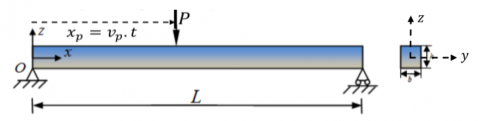Figure 1. FGM beam under a dynamic moving laod

The material properties of FGM vary continuously in the direction of the thickness (h) due to the progressive variation of the volume fraction of the constituent materials.

## 2.jpg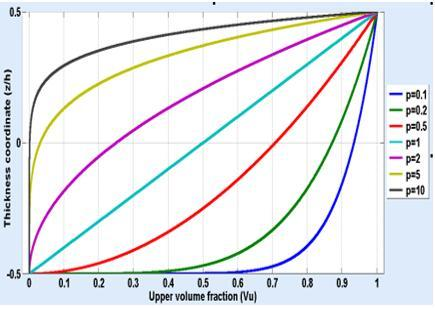Figure 2. Upper volume fraction profile (Vu) through the thickness of FGM beam

Based on the mixing rule, the actual material properties P can be written as:

$P={{P}_{U}}{{V}_{U}}+{{P}_{L}}{{V}_{L}}$     (1)

PU, PL, VU and VL are the corresponding material properties and the volume fractions of the upper and the lower surfaces of the beam bound by:

${{V}_{U}}+{{V}_{L}}=1$      (2)

In our study, FGM profile of the higher volume fraction is supposed to follow the shape of power law that is written by Wakashima et al. :

${{V}_{U}}={{\left( \frac{z}{h}+\frac{1}{2} \right)}^{p}}$      (3)

(p) is the power law index, non-negative constant (0≤p≤∞), which determines the mixing law variation along the thickness of the beam, as shown in Figure 2.

2.2 Displacement field

Based on the general refined shear deformation beam theory, the displacements coordinate of any point of the beam are given as:

$\left\{ \begin{matrix} U(x,z,t)={{u}_{o}}(x,t)-z.{{w}_{b,x}}(x,t)-f(z).{{w}_{s,x}}(x,t) \\ W(x,z,t)={{w}_{b}}(x,t)+{{w}_{s}}(x,t)\,\,\,\,\,\,\,\,\,\,\,\,\,\,\,\,\,\,\,\,\,\,\,\,\,\,\,\,\,\,\,\,\,\,\,\,\,\,\,\,\,\,\,\,\,\, \\\end{matrix} \right.$        (4)

## 3.jpg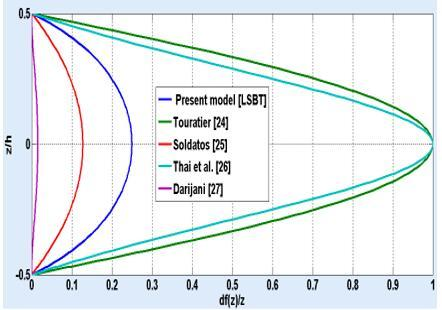Figure 3. Shear strain shape function of various beam models

uo is the axial displacement in the middle of the beam section. wb and ws are the components of transverse displacement in bending and shearing respectively of the average plane of the beam. f(z) is the shape function which characterizes the transverse shear and stress distribution along the thickness of the beam. The test function is chosen to satisfy the boundary conditions of the shear stresses on the upper and lower surfaces of the beam where the shear correction factor is not necessary. Consequently, the present model is defined by a refined logarithmic shear function (LSBT) identified by:

$f(z)=\frac{3h}{8}\ln \left( \frac{h-z}{h+z} \right)$    (5)

The partition of the transverse displacement in the parts in bending and in shearing helps to see the contributions due to shearing and the bending of the total transverse displacement. In the small disturbances hypothesis, the strain-displacement relations of the general beam theories are written as follows:

$\left\{ \begin{matrix} {{\varepsilon }_{xx}}={{u}_{o,x}}-z.{{w}_{b,xx}}-f(z).{{w}_{s,xx}}\,\,\,\,\,\, \\ {{\gamma }_{xz}}=(1-f{{(z)}_{,z}}).{{w}_{s,x}}=g(z).{{w}_{s,x}} \\\end{matrix} \right.$     (6)

g(z) is the function of the transverse refined shear deformation. Figure 3 illustrates the shape function of the transverse shear stress of different models. We show that the stress distribution of transverse shear is approximately parabolic, Thus, the conditions meet zero shear stress on the upper and lower surfaces of the beams [24-27].

2.3 Equations of motion

To derive the equations of motion, the equilibrium equations are obtained from the Hamilton principle. The principle can be stated in analytical from:

$\int_{{{t}_{1}}}^{{{t}_{2}}}{(\delta \prod -\delta L}+\delta V)$     (7)

t1 and t2 are the initial and final time, respectively; δΠ is the virtual variation of the strain energy; $\delta L$ is the virtual variation of the kinetic energy; and δV is the virtual variation of the work done by external forces :

The energy of virtual deformation δΠ:

$\delta \prod =\int_{V}{{{\sigma }_{ij}}\delta {{\varepsilon }_{ij}}dV=\int\limits_{V}{{{\sigma }_{xx}}\delta {{\varepsilon }_{xx}}dV}}+\int\limits_{V}{{{\sigma }_{xz}}\delta {{\gamma }_{xz}}dV}$     (8)

$\delta \prod =\int_{0}^{L}{(}N.\delta {{u}_{o,x}}-{{M}^{b}}.\delta {{w}_{b,xx}}-{{M}^{s}}.\delta {{w}_{s,xx}}+Q.\delta {{w}_{s,x}}).dx$   (9)

N, Mb, Ms and Q are the results of the constraints defined by:

$(N,{{M}^{b}},{{M}^{s}},Q)=\int\limits_{A}{{{\sigma }_{xx}}.(1,z,f(z),g(z)).dA}$      (10)

The results indicated with an exponent 'b' are the conventional bending moment of the classical beam theory; while the others with exponent ’s’ are additional quantities incorporating the effect of shear deformation. By substituting the stress-strain relationships for the definitions of force and at the moment resulting from current theory, we obtain the following constitutive equations:

$\left\{ \begin{matrix} N={{A}_{11}}.{{u}_{o,x}}-{{B}_{11}}.{{w}_{b,xx}}-{{E}_{11}}.{{w}_{s,xx}} \\ {{M}^{b}}={{B}_{11}}.{{u}_{o,x}}-{{D}_{11}}.{{w}_{b,xx}}-{{F}_{11}}.{{w}_{s,xx}} \\ {{M}^{s}}={{E}_{11}}.{{u}_{o,x}}-{{F}_{11}}.{{w}_{b,xx}}-{{H}_{11}}.{{w}_{s,xx}} \\ Q={{A}_{55}}.{{w}_{s,x}}\,\,\,\,\,\,\,\,\,\,\,\,\,\,\,\,\,\,\,\,\,\,\,\,\,\,\,\,\,\,\,\,\,\,\,\,\,\,\,\,\,\,\,\,\,\,\,\,\,\, \\\end{matrix} \right.$      (11)

Such as:

$({{A}_{11}},{{B}_{11}},{{D}_{11}},{{E}_{11}},{{F}_{11}},{{H}_{11}})=\int\limits_{A}{{{Q}_{11}}(z).}(1,z,f(z),{{z}^{2}},zf(z),f{{(z)}^{2}}).dA$     (12)

${{A}_{55}}=\int\limits_{A}{{{Q}_{55}}(z).[g{{(z)}^{2}}].dA}$     (13)

The reduced elastic constants are defined by:

${{Q}_{11}}(z)=\frac{E(z)}{(1-\nu {{(z)}^{2}})};\,\,{{Q}_{55}}(z)=\frac{E(z)}{2(1+\nu (z))}$      (14)

E(z) and v(z) are the Young's modulus and the Poisson's ratio of FGM beams respectively, which vary continuously in the direction of the axis "z" in accordance with the power law function P-FGM.

Including the effect of rotational and axial inertia, the virtual kinetic energy $\delta L$ can be expressed as:

$\delta L=\int\limits_{V}{\rho (z).(\dot{U}.\delta }\dot{U}+\dot{W}.\delta \dot{W}).dV$      (15)

\begin{align} & \delta L=\int_{0}^{L}{({{I}_{1}}.{{{\ddot{u}}}_{0}}}-{{I}_{2}}.{{{\ddot{w}}}_{b,x}}-{{I}_{3}}.{{{\ddot{w}}}_{s,x}}).\delta {{u}_{0}}.\delta x-\int_{0}^{L}{({{I}_{2}}.{{{\ddot{u}}}_{0}}}-{{I}_{4}}.{{{\ddot{w}}}_{b,x}}-{{I}_{5}}.{{{\ddot{w}}}_{s,x}}).\delta {{w}_{b,x}}.\delta x \\ & \,\,\,\,\,-\int_{0}^{L}{({{I}_{3}}.{{{\ddot{u}}}_{0}}}-{{I}_{5}}.{{{\ddot{w}}}_{b,x}}-{{I}_{6}}.{{{\ddot{w}}}_{s,x}}).\delta {{w}_{s,x}}.\delta x+\int_{0}^{L}{({{I}_{1}}({{{\ddot{w}}}_{b}}+{{{\ddot{w}}}_{s}}}).(\delta {{{\ddot{w}}}_{b}}+\delta {{{\ddot{w}}}_{s}}).\delta x \\\end{align}      (16)

Such as:

$({{I}_{1}},{{I}_{2}},{{I}_{3}}{{I}_{4}}{{I}_{5}}{{I}_{6}})=\int\limits_{A}{\rho (z).(1,z,f(z),{{z}^{2}},zf(z),f{{(z)}^{2}}}).dA$       (17)

The virtual potential energy δV of the mobile transverse charge at any time 't' is given below:

$\delta V=-\int_{0}^{L}{P(x,t).\delta ({{w}_{b}}+{{w}_{s}}}).dx$       (18)

$P(x,t)=P(t).\delta (x-{{x}_{p}})$      (19)

where,

$P(t)={{P}_{0}}.sin(\Omega .t)$       (20)

Po is the intensity of the concentrated load, xp is the coordinates of the mobile load, δ (.) Is the Dirac-delta function and Ω it’s the excitation frequency of the mobile harmonic load of FGM beams. By replacing the Eqns. (9), (16) and (18) in Eq. (7), by integrating by parts and obtains the following equations of equilibrium:

$\delta {{u}_{0}}:\,\,\,\,\,\,\,\,\,\,\frac{\partial N}{\partial x}={{I}_{1}}.{{\ddot{u}}_{0}}-{{I}_{2}}.{{\ddot{w}}_{b,x}}-{{I}_{3}}.{{\ddot{w}}_{s,x}}$      (21)

\begin{align} & \delta {{w}_{b}}:\,\,\,\,\,\,\,\,\,\,\frac{{{\partial }^{2}}{{M}^{b}}}{\partial {{x}^{2}}}+P(t).\delta (x-{{x}_{p}})={{I}_{2}}.{{{\ddot{u}}}_{0,x}}-{{I}_{4}}.{{{\ddot{w}}}_{b,xx}}-{{I}_{5}}.{{{\ddot{w}}}_{s,xx}} \\ & +{{I}_{1}}({{{\ddot{w}}}_{b}}+{{{\ddot{w}}}_{s}}) \\\end{align}    (22)

\begin{align} & \delta {{w}_{s}}:\,\,\,\,\,\,\,\,\,\,\frac{{{\partial }^{2}}{{M}^{s}}}{\partial {{x}^{2}}}+\frac{\partial Q}{\partial x}+P(t).\delta (x-{{x}_{p}})={{I}_{3}}.{{{\ddot{u}}}_{0}}-{{I}_{5}}.{{{\ddot{w}}}_{b,x}}-{{I}_{6}}.{{{\ddot{w}}}_{s,xx}} \\ & +{{I}_{1}}({{{\ddot{w}}}_{b}}+{{{\ddot{w}}}_{s}}) \\\end{align}      (23)

2.4 Mathematical resolution

The Rayleigh-Ritz method is adopted to discretize the partial derivatives of the displacement components of the forced vibration system and the form functions are developed in terms of the algebraic polynomial series as indicated by the following formulas.

\begin{align} & {{u}_{0}}(x,t)=\sum\limits_{j=1}^{n}{{{\varphi }_{j}}(x).u_{j}^{0}(t)};\,\,\,\,{{w}_{b}}(x,t)=\sum\limits_{k=1}^{n}{{{\psi }_{k}}(x).w_{k}^{b}(t);\,\,} \\ & {{w}_{s}}(x,t)=\sum\limits_{p=1}^{n}{{{\phi }_{p}}(x).w_{p}^{s}(t)} \\\end{align}        (24)

After performing the integration by part on Eqns. (21), (22) and (23) with the weighted functions respectively φi(x), Ψi(x) and ϕi(x)(i=1,2,...n), which must satisfy the boundary conditions, the weak forms of government equations of motion, equivalent to both ordinary differential equations can be written in the following final form:

$[M]\left\{ \left. \ddot{q}(t) \right\} \right.+[K]\left\{ \left. q(t) \right\} \right.=\left\{ \left. F(t) \right\} \right.$     (25)

[M] and [K] are the mass and rigidity matrices respectively, their order is [3n×3n], {q (t)} is the column vector of unknown coefficients temporary, of the order [3n×1]. {F(t)} is the generalized vector produced by the transverse load in motion of the order [3n×1].

3. Numerical Results

We are to present a set of results obtained following the execution of Matlab calculation program, which is based on the mathematical development of a forced vibration model, actuated by a mobile harmonic loads on the FGM beams, under varying parameters with arbitrarily boundary conditions, i.e., Simply–Simply (S–S), Clamped–Simply (C–S), Clamped–Clamped (C–C), and Clamped–Free (C–F).

The natural frequencies are determined by solving the system of equations governing the eigenvalue problems. The dynamic responses of the moving harmonic loads are calculated numerically using Newmark's method in the critical section of the FGM beams, where the deformations are maximum. For the desired precision in the calculation, the time is subdivided into 400 stations with a uniform time increment (Δt). The functionally graded materials (FGMs) consist of a mixture for ceramic and metal whose properties vary continuously through the thickness direction of the beams according to the power law function (P-FGM). The upper part of the beams (z=+h/2) is purely ceramic (PU=100% Alumina), while the lower part of the beams (z=-h/2) is purely metal (PL=100% Aluminum).

The mechanical properties of Alumina (Al2O3) are: Ec=380GPa, ρ=3960 Kg/m3, v=0.3, and those of Aluminum (Al) are: Em=70 Gpa, ρ=2702 Kg/m3, v=0.3. The indices of the Rayleigh-Ritz test function which must satisfy the boundary conditions are given in Table 1 .

Table 1. Admisible functions index for random boundary conditions

 BCs P0 q0 C–C C–S C–F S–S 2 2 2 1 2 1 0 1

For practical reasons, the natural frequencies $({{\varpi }_{n}})$ , the velocity of the mobile load (α) and the excitation frequency of the moving harmonic force (Ω), which is represented by the frequency ratio (β), are defined by dimensionless parameters respectively, as follows:

${{\varpi }_{n}}=\frac{{{\omega }_{n}}{{L}^{2}}}{h}\sqrt{\frac{\rho L}{{{E}_{L}}}};\,\,\,\,\,\,\,\,\,\,\,\,\,\,\,\,\,\,\,\,\,\alpha =\frac{{{\nu }_{p}}}{{{\nu }_{cr}}};\,\,\,\,\,\,\,\,\,\,\,\,\,\,\,\,\,\,\,\,\,\beta =\frac{\Omega }{{{\omega }_{1}}}$

where vcr  is the critical quantity of mobile charging speed determined by Fryba . ω1 is the first fundamental frequencies of the FGM beams. The direction of the moving load is from left to right. Therefore, when t = 0, the point force (P) is in the left support of the beam (xp=0), when t=L/vp, the point force (P) arrived at the right support of the beam (xp=L). The Dynamic Amplification Factor (DAF) is determined by:

$\mathrm{DAF}=\frac{\mathrm{W}_{\mathrm{Dynamic}, \max }}{\mathrm{W}_{\mathrm{Static}, \max }}$

wDynamic,max and wStatic,max correspond respectively to the dynamic transverse deflection and to the static deflection, on the critical section of the FGM beams which gives a maximum transverse displacement.

In Table 2, a variation of the first nondimensional fundamental frequency ($\varpi_{1}$) is performed using the present logarithmic LSBT model. The study is done while changing the power low parameter (p) of the FG material, for various boundary conditions of the beam (S-S, C-S, C-C and C-F) with L/h=20. The results in this table are presented without taking into account the effect of the Poisson's ratio (υ) in the expression of the reduced stiffness coefficient (Q11). The comparison shows an excellent agreement between the numerical results and those Nguyen et al. , which approve the accuracy of our proposed model.

Table 2. Eigenfrequencies ($\varpi_{1}$) of the FGM beams

 BCs Model Power low exponent (p) 0 1 2 5 10 ∞ S-S Present Ref.  5.4611 5.4603 4.5028 4.2051 4.2687 3.8361 4.0073 3.6485 3.7393 3.5390 2.8376 --- C-S Present Ref.  8.4880 --- 6.6534 --- 6.1297 --- 5.8011 --- 5.5666 --- 4.4103 --- C-C Present Ref.  12.2398 12.2243 9.4919 9.4319 8.6785 8.5977 8.2187 8.1446 7.9353 7.8860 6.3597 --- C-F Present Ref.  1.9498 1.9496 1.5073 1.5011 1.3782 1.3696 1.3107 1.3034 1.2689 1.2646 1.0131 ---

Table 3. Dynamic Amplification Factor (DAF) of the FGM beams

 BCs Model Power low exponent (p) 0 1 3 4 5 S-S LSBT FSBT CBT 1.8682 1.8674 1.8835 1.8680 1.8672 1.8825 1.8680 1.8672 1.8825 1.8661 1.8658 1.8821 1.8661 1.8658 1.8821 C-S LSBT FSBT CBT 1.6171 1.6165 1.6013 1.6172 1.6166 1.6038 1.6199 1.6185 1.6053 1.8616 1.8632 1.8827 1.6171 1.6165 1.6013 C-C LSBT FSBT CBT 1.5245 1.5236 1.5370 1.5258 1.5250 1.5370 1.5257 1.5251 1.5371 1.6252 1.6208 1.6028 1.5245 1.5236 1.5370 C-F LSBT FSBT CBT 1.3805 1.3806 1.3684 1.3777 1.3778 1.3684 1.3779 1.3774 1.3684 1.5231 1.5227 1.5370 1.3805 1.3806 1.3684

The fundamental frequencies are inversely proportional to the power exponent, the decrease of the parameter (p) gives rise to an increase in flexural rigidity. So, it can be said that the change of the material constitutes a significant effect on the results of the free vibration of FGM beams. We also note that the rate of variation of the frequencies increases when the rigidity of the beam increases, while change the parameter of power (p). The lowest rate of the eigenfrequencies is given by the condition of supports C-F and the rate the highest is given by the C-C support condition. This implies that the influence of the power exponent (p) on the fundamental frequency variation is important for low flexibility beams.

Table 3 shows the variation of Dynamic Amplification Factor (DAF) of the FGM beams with different modes of support (S-S, C-S, C-C and C-F), by varying the power exponent (p). This study is modeled by using different orders of the beams theories at α=0.2, β=0.5, and L/h=5. The shear correction factor is considered as ks = 5/6 for FSBT. It should be noted that the Dynamic Amplification Factor varies depending on the applied boundary condition. The power parameter effect (p) on the (DAF) is less obvious on all boundary conditions. For homogeneous beam (k=0 or k=∞), the (DAF) take the same value.

We also note that in S-S and C-C boundary conditions, the DAF calculated by taking account of the warping effect (FSBT and LSBT) is relatively lesser compared to that calculated with classical Euler-Bernoulli theory (CBT) , is relatively superior for both C-S  and C-F support conditions; the variation rate of DAF in this boundary condition is higher compared to the S-S and C-C limit conditions, while the two theories FSBT and LSBT give substantially the same values for general modes of support. From there, we conclude that the transverse shearing effect should be considered in the mathematical modeling to calculate the Dynamic Amplification Factor (DAF) of the FGM beam.

Figure 4 describes the relationship between the speed of the mobile harmonic forces (α) and the Dynamic Amplification Factor (DAF) to the critical displacement of the FGM beams, for a frequencies ratio  β= 0.1, 0.5, 1 and 1.5 , under arbitrarily boundary conditions (S-S, C-S, C-C and C-F), with p=2 and L/h=10. It is evident that the velocity of the mobile charge (α) plays a major role in the variation of the Dynamic Amplification Factor. The DAF results of the low excitation frequency of the harmonic mobile load (β= 0.1) change in a regular form unlike the high harmonic moving load which change randomly. Except when β=1, the dynamic deflections increase generally until a certain value of the velocity parameter (α), after this critical value, a growth of the speed parameter causes a reduction on the DAF, with some local peaks in the transverse curves of the mobile harmonic charges. Once the velocity parameter (α) is set to 0, this is the case of the static deflection loaded with a concentrated force at the critical section of the FGM beams (DAF=1).

## 4a.pnga) β= 0.1

## 4b.png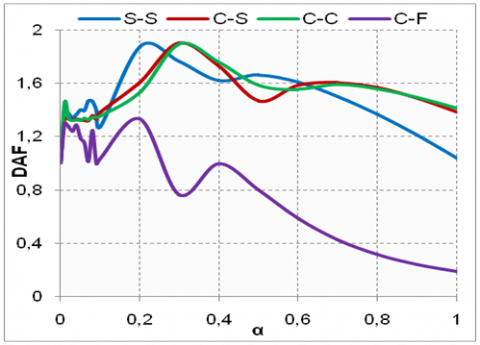b)  β= 0.5

## 4c.png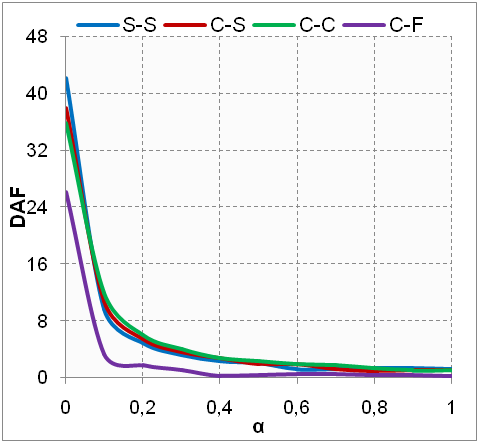c) β= 1

## 4d.png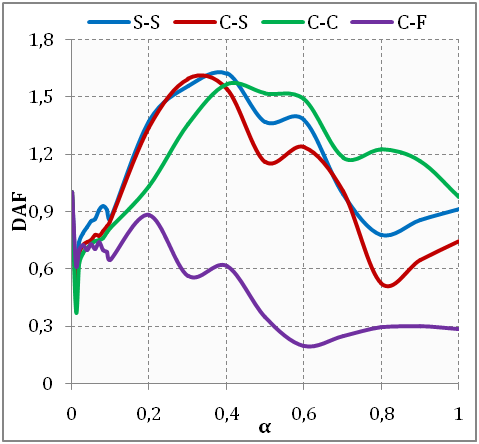d) β= 1.5

Figure 4. DAF variations of the FGM beams

In addition, the critical speed cited by Fryba  does not correspond to the maximum deflection of the beams, the highest rate for which a significant DAF changes as a function of the excitation frequencies β and the support mode applied. The DAF considerably affects on the S-S support condition relative to the C-S and C-C boundary conditions, and weakly affects to the cantilevers beam. The impact of the excitation frequencies on the DAF where β = 1 is very crucial when the charge moves at low speed. After this passage, the resonance phenomenon disappears and the Dynamic Amplification Factor returns to its normal value for all the boundary conditions. For the low excitation frequencies ratio (β≈0), the maximum dynamic deflection becomes less than the maximum static deflection (DAF <1), whatever the speed of the moving harmonic force exerted.

4. Conclusion

In this research, the dynamic behavior of functionally graded beams (FGMs) transported by harmonics moving forces was analyzed, under arbitrarily boundary conditions. First, we studied the fundamental frequencies of free vibration problem to describe the critical velocities of the moving loads, using a new distribution of the refined logarithmic transverse shear function (LSBT). This theory satisfies the zero traction boundary conditions on the top and bottom surfaces of FGM beam without shear correction factors. Secondly, we focused on the numerical solutions obtained from the mathematical formulation of forced vibration behavior for all orders of the beams theories. A parametric study was done to analyze in detail the impact of different parameters such as; the material distribution, the transverse shear deformation effect, the velocities and the excitation frequencies of the moving harmonic load on modal responses. We can affirm that the present dynamic model is not only accurate, but is also effective for analyzing the forced vibration of the FGMs beams excited by moving harmonic loads, regardless of the physical and geometrical conditions applied.

Nomenclature
 L Length of beam h Thickness of the beam b Width of beam P Concentrated harmonic force P0 Intensity of the concentrated loads, p Power index E Young's module vp Constant speed, ν Poisson coefficient I inertia term $\sigma$ Normal stress, $\varepsilon$ Normal strain $\gamma$ Shear strain, rad $u_{0}$ Axial Displacement along, $\mathcal{W}_{b}$ Transverse displacement in bending, $\mathcal{W}_{s}$ Transverse displacement in shearing, Greek symbols δV virtual potential energy $\delta L$ virtual kinematic energy $\delta \prod$ virtual deformation energy ks The shear correction factor b Frequencies ratio $\rho$ Mass density, Kg.m-3 α Velocity of the mobile load δ(.) Dirac-delta function Ω Excitation frequency of the mobile harmonic load $\omega$ Eignfrequency of the FGM beam $\varpi$ Nondimensional fundamental frequency Q Reduced stiffness coefficient
References

 Daikh, A.A. (2018). Vibration libre et flambement des structures sandwichs en FGM (Doctoral dissertation). Université Djillali Liabes (Sidi Bel Abbes), Algerie.

 Sun, Y., Li, S., Batra, R. (2016). Thermal buckling and post-buckling of FGM Timoshenko beams on nonlinear elastic foundation. Journal of Thermal Stresses, 39(1): 11-26. https://doi.org/10.1080/01495739.2015.1120627

 Lee, Y., Bae, S., Kim, J. (2016). Thermal buckling behavior of functionally graded plates based on neutral surface. Composite Structures, 137: 208-214. https://doi.org/10.1016/j.compstruct.2015.11.023

 Akavci, S. (2016). Mechanical behavior of functionally graded sandwich plates on elastic foundation. Composites Part B: Engineering, 96: 136-152. https://doi.org/10.1016/j.compositesb.2016.04.035

 Şimşek, M. (2016). Buckling of Timoshenko beams composed of two-dimensional functionally graded material (2D-FGM) having different boundary conditions. Composite Structures, 149: 304-314. https://doi.org/10.1016/j.compstruct.2016.04.034

 Elhannani, A., Refassi, K., Elmeiche, A., Bouamama, M. (2019). Vibration analysis of functionally graded tapered rotor shaft system. Mechanics and Mechanical Engineering, 23(1): 241-245. https://doi.org/10.2478/mme-2019-0032

 Elmeiche, A., Megueni, A., Lousdad, A. (2016). Free vibration analysis of functionally graded Nanobeams based on different order beam theories using Ritz method. Periodica Polytechnica Mechanical Engineering, 60(4): 209-219. http://dx.doi.org/10.3311/PPme.8707

 Hassan, A.H.A., Kurgan, N. (2019). A review on buckling analysis of functionally graded plates under thermo-mechanical loads. International Journal of Engineering & Applied Sciences, 11(1): 345-368. http://dx.doi.org/10.24107/ijeas.555719

 Ebrahimi, F., Ghasemi, F., Salari, E. (2016). Investigating thermal effects on vibration behavior of temperature-dependent compositionally graded Euler beams with porosities. Meccanica, 51(1): 223-249. https://doi.org/10.1007/s11012-015-0208-y

 Leissa, A. (1978). A direct method for analyzing the forced vibrations of continuous systems having damping. Journal of Sound and Vibration, 56(3): 313-324. https://doi.org/10.1016/S0022-460X(78)80150-3

 Zaoui, F.Z., Hanifi, H.A., Abderahman, L.Y., Mustapha, M.H., Abdelouahed, T., Djamel, O. (2017). Free vibration analysis of functionally graded beams using a higher-order shear deformation theory. Mathematical Modelling of Engineering Problems, 4(1): 7-12. https://doi.org/10.18280/mmep.040102

 Kirs, M., Karjust, K., Aziz, I., Õunapuu, E., Tungel, E. (2018). Free vibration analysis of a functionally graded material beam: Evaluation of the Haar wavelet method. Proceedings of the Estonian Academy of Sciences, 67(1): 1. https://doi.org/10.3176/proc.2017.4.01

 Kapuria, S., Bhattacharyya, M., Kumar, A. (2008). Bending and free vibration response of layered functionally graded beams: A theoretical model and its experimental validation. Composite Structures, 82(3): 390-402. https://doi.org/10.1016/j.compstruct.2007.01.019

 Nejad, M., Hadi, A. (2016). Non-local analysis of free vibration of bi-directional functionally graded Euler–Bernoulli nano-beams. International Journal of Engineering Science, 105: 1-11. https://doi.org/10.1016/j.ijengsci.2016.04.011

 Asghari, M., Rahaeifard, M., Kahrobaiyan, M., Ahmadian, M. (2011). The modified couple stress functionally graded Timoshenko beam formulation. Materials & Design, 32(3): 1435-1443. https://doi.org/10.1016/j.matdes.2010.08.046

 Şimşek, M., Kocatürk, T., Akbaş, Ş. (2013). Static bending of a functionally graded microscale Timoshenko beam based on the modified couple stress theory. Composite Structures, 95: 740-747. https://doi.org/10.1016/j.compstruct.2012.08.036

 Bouamama, M., Elmeiche, A., Elhennani, A., Kebir, T. (2019). Dynamic stability analysis of functionally graded timoshenko beams with internal viscous damping distribution. Journal Européen des Systèmes Automatisés, 52(4): 341-346. http://dx.doi.org/10.18280/jesa.520402

 Rajabi, K., Kargarnovin, M., Gharini, M. (2012). Dynamic analysis of a functionally graded simply supported Euler–Bernoulli beam subjected to a moving oscillator. Acta Mechanica, 224(2): 425-446. http://dx.doi.org/10.1007/s00707-012-0769-y

 Foda, M., Abduljabbar, Z. (1998). A dynamic green function formulation for the response of a beam structure to a moving mass. Journal of Sound and Vibration, 210(3): 295-306. https://doi.org/10.1006/jsvi.1997.1334

 Nguyen, D.K., Thom, T.T., Gan, B.S., Van Tuyen, B. (2016). Influences of dynamic moving forces on the functionally graded porous-nonuniform beams. International Journal of Engineering and Technology Innovation, 6(3): 173-189. http://ojs.imeti.org/index.php/IJETI/article/view/149

 Panigrahi, B., Pohit, G. (2020). Modelling and analysis of functionally-graded cracked beams subjected to static and dynamic loadings. In Handbook of Research on Developments and Trends in Industrial and Materials Engineering (pp. 306-326). IGI Global. https://doi.org/10.4018/978-1-7998-1831-1.ch013

 Praharaj, R.K., Datta, N. (2020). Dynamic response spectra of fractionally damped viscoelastic beams subjected to moving load. Mechanics Based Design of Structures and Machines, 1-15. https://doi.org/10.1080/15397734.2020.1725563

 Wakashima, K., Hirano, T., Niino, M. (1990). Space applications of advanced structural materials. ESA SP, 303, 97.

 Touratier, M. (1991). An efficient standard plate theory. International Journal of Engineering Science, 29(8): 901-916. https://doi.org/10.1016/0020-7225(91)90165-Y

 Soldatos, K.P., Timarci, T. (1993). A unified formulation of laminated composite, shear deformable, five-degrees-of-freedom cylindrical shell theories. Composite Structures, 25(1-4): 165-171. https://doi.org/10.1016/0263-8223(93)90162-J

 Thai, C.H., Ferreira, A.J.M., Bordas, S.P.A., Rabczuk, T., Nguyen-Xuan, H. (2014). Isogeometric analysis of laminated composite and sandwich plates using a new inverse trigonometric shear deformation theory. European Journal of Mechanics-A/Solids, 43: 89-108. https://doi.org/10.1016/j.euromechsol.2013.09.001

 Darijani, H., Mohammadabadi, H. (2014). A new deformation beam theory for static and dynamic analysis of microbeams. International Journal of Mechanical Sciences, 89: 31-39. https://doi.org/10.1016/j.ijmecsci.2014.08.019

 Frýba, L. (1972). Vibration of solids and structures under moving loads. Noordhoff International, Groningen, The Netherlands. https://doi.org/10.1007/978-94-011-9685-7